Question

# Today you purchase a coupon bond that pays an annual interest, has a par value of...

Today you purchase a coupon bond that pays an annual interest, has a par value of \$1,000, matures in six years, has a coupon rate of 10%, and has a yield to maturity of 8%. One year later, you sell the bond after receiving the first interest payment and the bond's yield to maturity had changed to 7%. Your annual total rate of return on holding the bond for that year is ?

Price=Par value*Coupon rate/yield*(1-1/(1+yield)^t)+Par value/(1+yield)^t

Purchase Price=1000*10%/8%*(1-1/1.08^6)+1000/1.08^6=1092.457593

Selling Price=1000*10%/7%*(1-1/1.07^5)+1000/1.07^5=1123.005923

Total rate of return=(1123.005923+1000*10%)/1092.457593-1=11.95%

#### Earn Coins

Coins can be redeemed for fabulous gifts.

Similar Homework Help Questions
• ### 1. Which of the following statement is correct about systematic risk and non-systematic risk? A. Systematic...

1. Which of the following statement is correct about systematic risk and non-systematic risk? A. Systematic risk can be eliminated by proper diversification. B. Fluctuation in oil price is a non-systematic risk. C. Financial markets reward you for bearing systematic risk. D. A stock’s systematic risk is measured by the standard deviation of its return. 2. As discussed in class, based on the CAPM, an electric utility will have the greater cost of equity capital than an airline company. True...

• ### A coupon bond that pays interest annually has a par value of \$1,000, matures in six...

A coupon bond that pays interest annually has a par value of \$1,000, matures in six years, and has a yield to maturity of 11%. The intrinsic value of the bond today will be ________ if the coupon rate is 7.5%. A) \$886.28 B) \$851.93 C) \$1,123.01 D) \$1,000.00 E) \$712.99

• ### A coupon bond pays annual interest, has a par value of \$1,000, matures in 5 (five)...

A coupon bond pays annual interest, has a par value of \$1,000, matures in 5 (five) years, has a coupon rate of 7.45%, and has a yield to maturity of 8.82%. The current yield on this bond is ________%. (2 decimal place)

• ### 4. A coupon bond that pays interest semi-annually has a par value of \$1,000, matures in...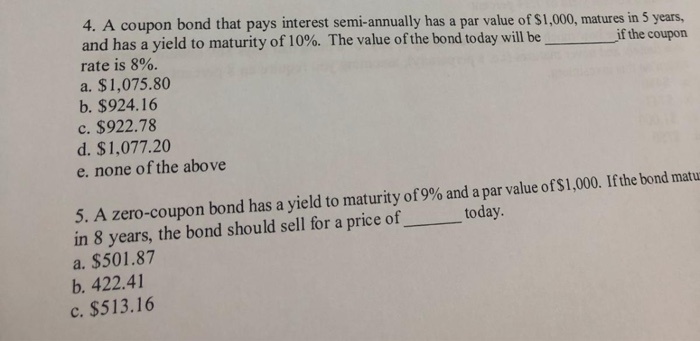4. A coupon bond that pays interest semi-annually has a par value of \$1,000, matures in 5 years, and has a yield to maturity of 10%. The value of the bond today will be rate is 8% a. \$1,075.80 b.\$924.16 if the coupon c. \$922.78 d. \$1,077.20 e. none of the above 5. A zero-coupon bond has a yield to maturity of 9% and a par value of\$1,000. Ifthe bond matu in 8 years, the bond should sell for a...

• ### Question 7 2 pts A coupon bond pays annual interest, has a par value of \$1,000,...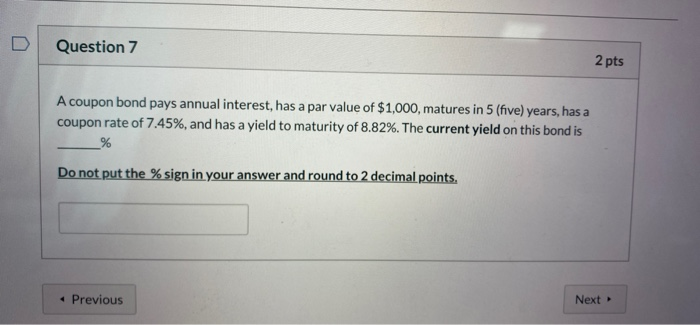Question 7 2 pts A coupon bond pays annual interest, has a par value of \$1,000, matures in 5 (five) years, has a coupon rate of 7.45%, and has a yield to maturity of 8.82%. The current yield on this bond is % Do not put the % sign in your answer and round to 2 decimal points. Previous Next

• ### You own a bond that has a 6% annual coupon rate and matures 5 years from...You own a bond that has a 6% annual coupon rate and matures 5 years from now. You purchased this 10-year bond at par value when it was originally issued. Which one of the following statements applies to this bond if the relevant market interest rate is now 5.8% (yield to maturity)? You purchase a bond with a coupon rate of 6.25% and a par value of \$1,000. There are 53 days to the next semiannual coupon payment date and...

• ### 6. Suppose that you purchase a 2 year coupon bond at the time it is issued...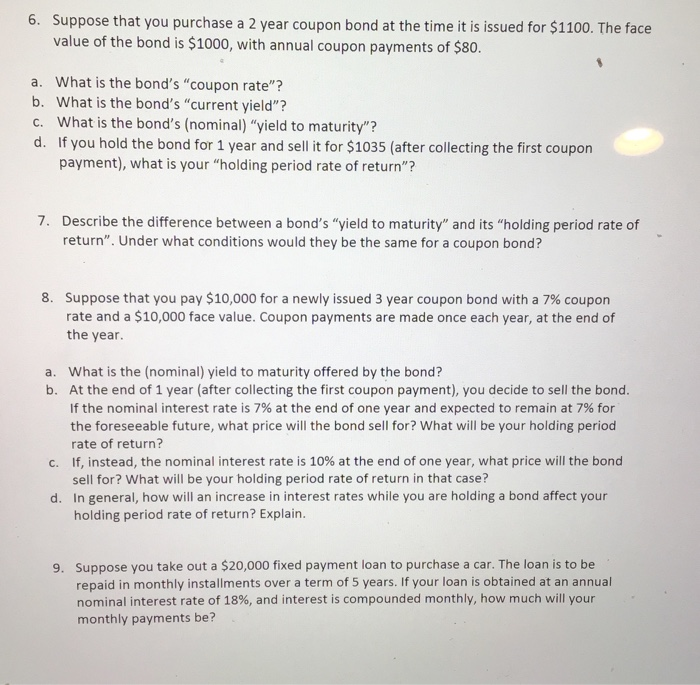6. Suppose that you purchase a 2 year coupon bond at the time it is issued for \$1100. The face value of the bond is \$1000, with annual coupon payments of \$80. a. What is the bond's "coupon rate"? b. What is the bond's "current yield"? C. What is the bond's (nominal) "yield to maturity"? d. If you hold the bond for 1 year and sell it for \$1035 (after collecting the first coupon payment), what is your "holding period...

• ### A coupon bond that pays interest annually has a par value of \$1000, matures in 11...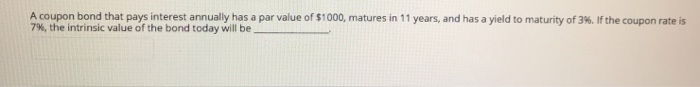A coupon bond that pays interest annually has a par value of \$1000, matures in 11 years, and has a yield to maturity of 3%. If the coupon rate is 7%, the intrinsic value of the bond today will be

• ### A coupon bond that pays interest semiannually has a par value of \$1000, matures in 9...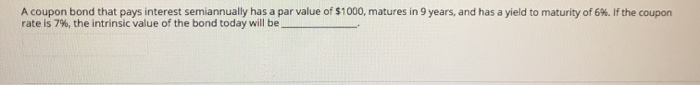A coupon bond that pays interest semiannually has a par value of \$1000, matures in 9 years, and has a yield to maturity of 6%. If the coupon rate is 7%, the intrinsic value of the bond today will be

• ### A coupon bond that pays interest annually has a par value of \$1000, matures in 11...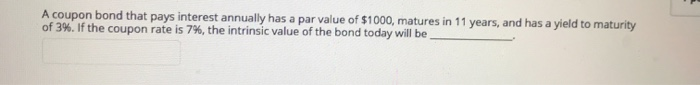A coupon bond that pays interest annually has a par value of \$1000, matures in 11 years, and has a yield to maturity of 3%. If the coupon rate is 7%, the intrinsic value of the bond today will be